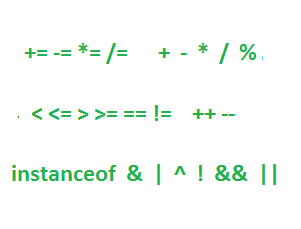# Java Operators

## 1. Compound Assignment Operators

The most commonly used compound assignment operators are +=, -=, *=, %=, and /=.

#### Example 1.1 Compound Assignment Operators

``````a -= 9;
b += 4 * 9;``````

## 2. Relational Operators

• The most commonly used relational operators are: <, <=, >, >=, == and !=.
• Relational operators always result in a boolean value.
• When comparing characters, Java uses the Unicode value of the character as the numerical value.
• Equality operators are == equals and != not equals.
• Numbers, characters, booleans, and reference variables can be tested with equality operators.
• If reference variables are compared, == returns true only if both references refer to the same object.
• Only compatible types can be compared.
• It is possible to use either == operator or equals() method to compare enum.

## 3. The instanceof

The instanceof operator is used to verify whether an object is of a particular type. And it is used only for object reference variables.

#### Example 3.1 Usage of instanceof

``````String str = "OCPJP 8";
if (str instanceof String) {
System.out.print("str is a String");
}``````
• The instanceof operator can be used only to test objects against class types that are in the same class hierarchy. It is illegal to use the instanceof operator to test across two different class hierarchies.
• For interfaces, an object passes the instanceof test if any of its superclasses implement the interface on the right side of the instanceof operator.
• It is legal to test if the null reference is an instance of a class, but it will always result in false.

## 4. Arithmetic Operators

• The basic arithmetic operators are + addition, – subtraction, * multiplication,  / division.
• The remainder operator (%), returns the remainder of a division.
• Expressions are evaluated from left to right, unless there are added parentheses, or unless some operators in the expression have higher precedence than others.
• The *, /, and % operators have higher precedence than + and -.

## 5. String Concatenation Operator

• If either operand is a String, the + operator concatenates the operands.
• If both operands are numeric, the + operator adds the operands.

## 6. Increment and Decrement Operators

• Prefix operators (++ and --) run before the value is used in the expression.
• Postfix operators (++ and --) run after the value is used in the expression.
• In any expression, both operands are fully evaluated before the operator is applied.
• Variables marked final cannot be incremented or decremented.

## 7. Conditional Operator

The conditional operator is a ternary operator (it has three operands), which is used to evaluate boolean expressions and assign a value to a variable.

#### Example 7.1 Conditional Operator Syntax

``x = (boolean expression) ? value to assign if true : value to assign if false``

## 8. Logical Operators

• The most commonly used "logical" operators are: &, |, ^, !, && and ||.
• Logical operators work with two expressions (except for !) that must resolve to boolean values.
• The short-circuit logical operators: && short-circuit AND , || short-circuit OR. They don't waste time on pointless evaluations.
• The && and & operators return true only if both operands are true.
• The || and | operators return true if either or both operands are true.
• The && operator does not evaluate the right operand if the left operand is false.
• The || does not evaluate the right operand if the left operand is true.
• The non-short-circuit logical operators & and | always evaluate both operands.
• The ^ operator (called the "logical XOR"), returns true if exactly one operand is true.
• The ! operator (called the "inversion" operator), returns the opposite value of the boolean operand it precedes.
Trustpilot